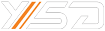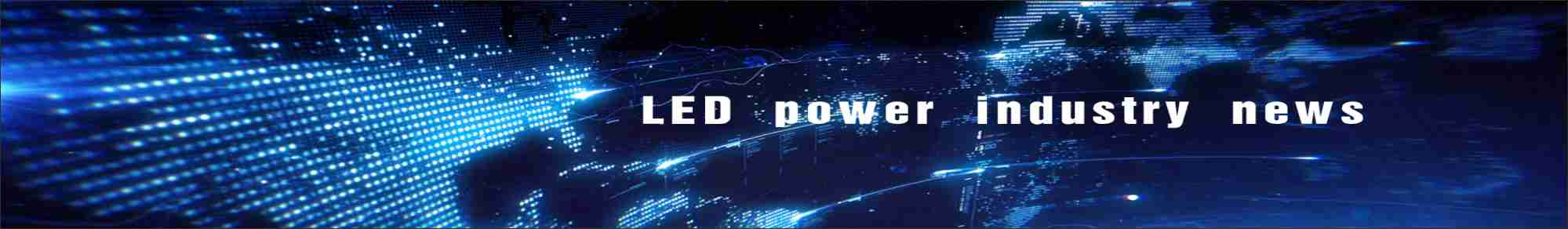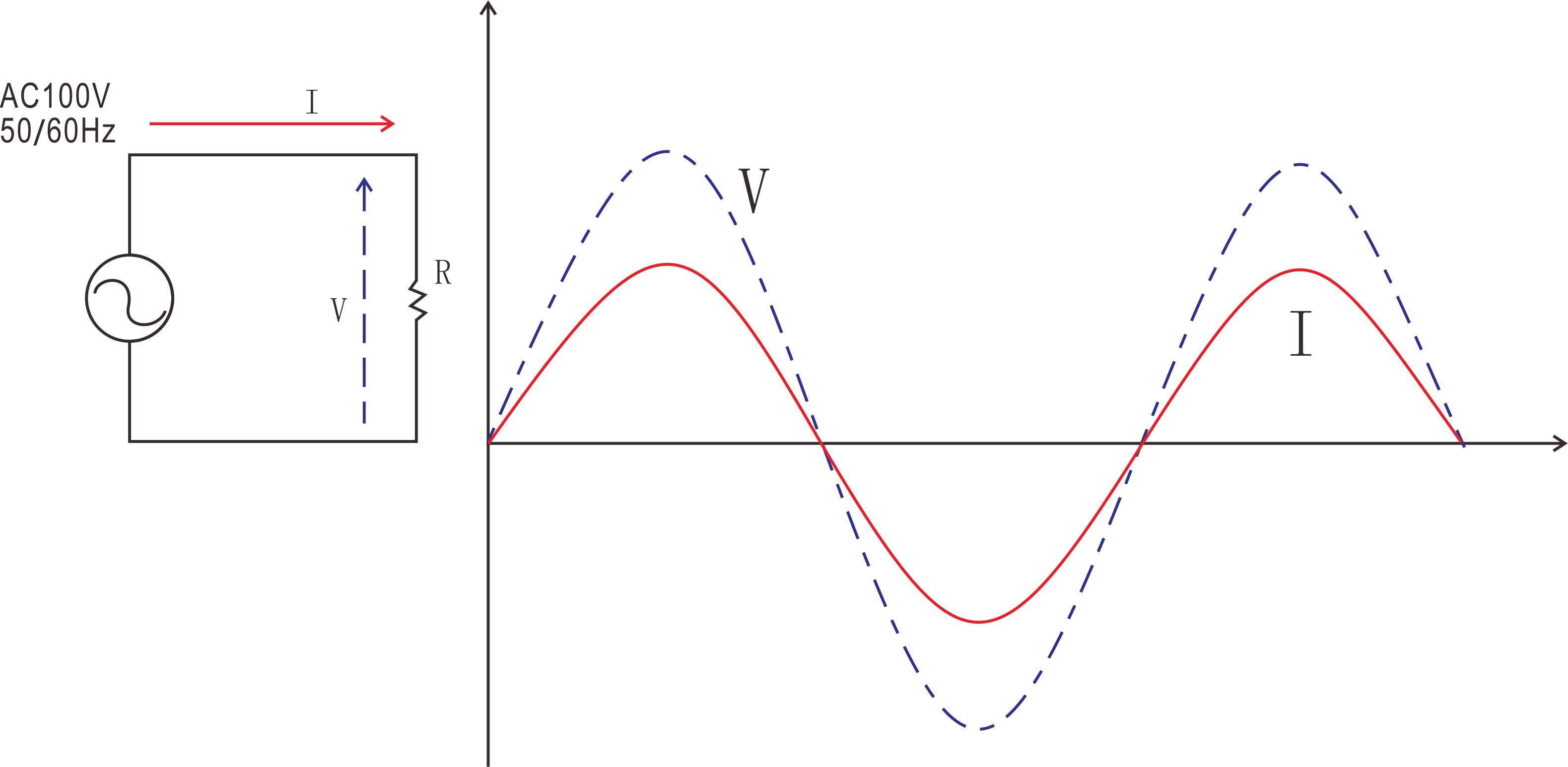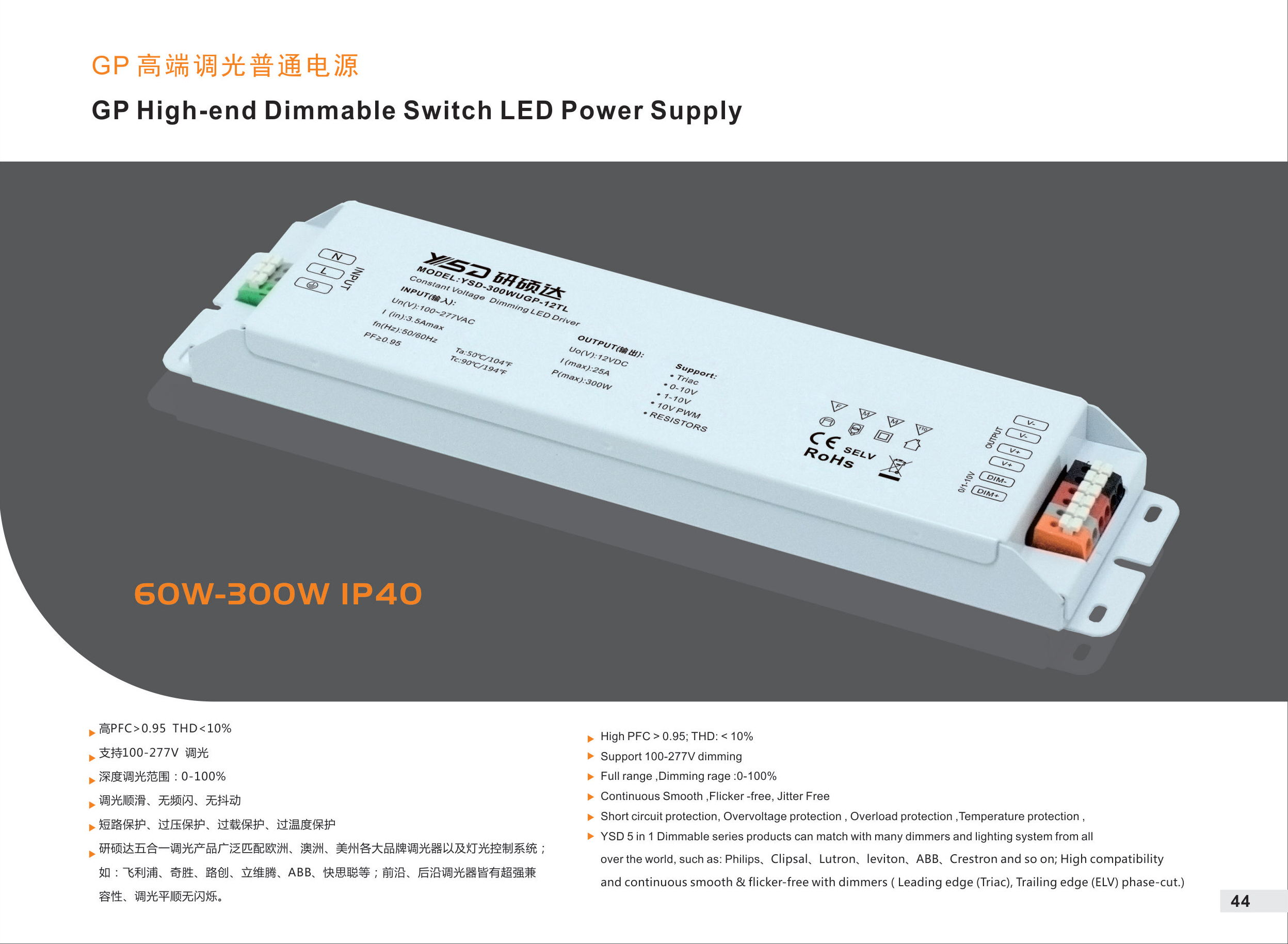#What is the power factor?What is the importance of the power factor?
Your Position：Home>News>Industry-news>What is the power factor?What is the importance of the power factor?

# What is the power factor?What is the importance of the power factor?

## What is the power factor?

Power factor refers to the ratio of the active power of the AC circuit to the apparent power.Under a certain voltage and power, the higher the value, the better the efficiency, and the more fully the power generation equipment can be utilized.

The size of the Power Factor is related to the load nature of the circuit, such as incandescent bulb, resistance furnace and other resistance load of Power Factor is 1, generally has the Power Factor of inductive load circuit is less than 1.Power factor is an important technical data of power system.Power factor is a coefficient to measure the efficiency of electrical equipment.Low power factor indicates that the reactive power of the circuit used for alternating magnetic field conversion is large, thus reducing the utilization rate of the equipment and increasing the power supply loss of the line.In AC circuits, the cosine of the phase difference between voltage and current (Φ) is called the power factor, expressed by the symbol cos N, in numerical terms, the power factor is the ratio of active power and apparent power, that is, cos D =P/S.Common nonlinear loads on power systems include rectifiers (used in power supplies), or devices such as fluorescent lamps, welders, or arc discharge furnaces.Because the current in these systems is interrupted by element switching, the current contains a harmonic component at a frequency that is an integer multiple of the power supply system.Distortion Power Factor can be used to measure the effect of harmonic Distortion on the mean Power of electric current.The chordal wave voltage and non - chordal wave current of computer power supply have a distortion power factor of 0.75.

## Switching power supply

Switching power supply is a common nonlinear load found in at least millions of personal computers in the world, with power output ranging from a few watts to a kilowatt.Early cheap with a full wave rectifier in switching power supply, rectifier only when the input voltage of the power supply more than the internal of capacitor voltage conduction, so its peak factor is high, the distortion power factor is low, and in the current of three-phase system, the linear current is not zero, there may be a neutral line load is too large.

A typical switching power supply first uses a rectifying diode to generate a DC voltage, which in turn generates an output voltage.Because the rectifier is a nonlinear element, its input current will have a lot of high harmonic components.This is a problem for power companies because they cannot compensate for the high frequency harmonics by adding capacitors and inductors.Therefore, some regions have started to legislate to require all power supply above a certain value of power factor correction function.

In order to improve the power factor, the European Union has set the harmonics standard.To comply with the current EU standard EN61000-3-2, all switching power supplies with an output power greater than 75W must have at least passive power factor correction (passive PFC) function.The 80W switching power supply certification requires the power factor to reach at least the level of 0.9.## What is the point of increasing the power factor?

1. Reduce the power loss of the line;

2. Reduce the voltage loss of the line;

3. Make full use of the active power of power supply equipment, improve the utilization rate of power supply equipment and reduce the cost of electric energy;

4. It can improve the quality of power supply and increase the utilization rate of power generation equipment;

## What are the measures to improve the power factor?

1. Adopt shunt phase-shifting capacitor compensation;

2. Improve the natural power factor;